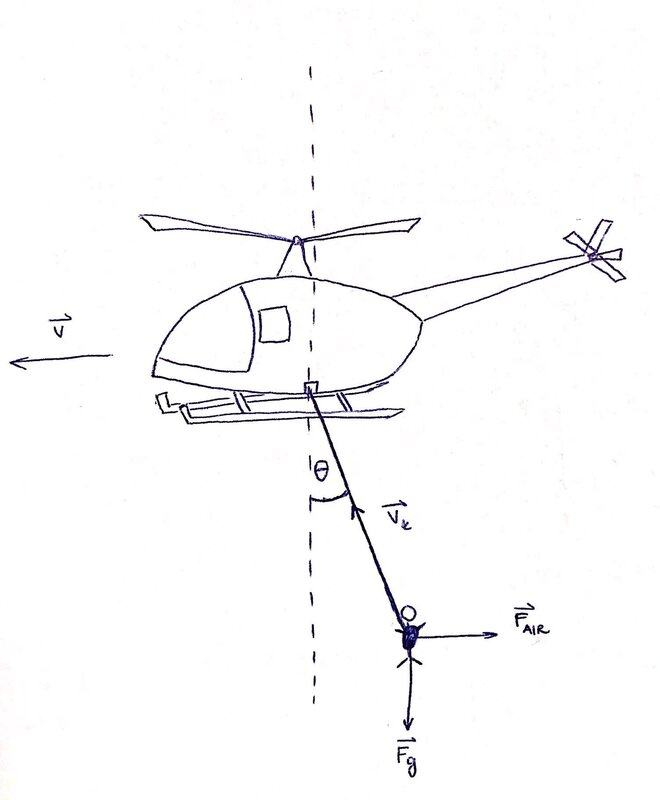# Lifting a survivor into a helicopter with a rope

bolzano95
Homework Statement:
A helicopter is flying horizontally with constant velocity ##v## and carries a survivor who hanging on a rope with length ##l##. Suddenly the pilot receives a message he has to pull the the survivor into the the helicopter as soon as possible. A paramedic on board starts to pull a hanging rope with such force that the length of rope is shortening with velocity ##v_k##.

What is the angle ##\theta## between the rope and vertical line?
Relevant Equations:
Relevant equations are below.We have 2 forces affecting the rope: 1. Gravitational force of the body ##=mg## and 2. Force of air = Force of drag= ##F_{AIR}##.
The length of the rope is shortening with the velocity ##v_k##.
So to figure out the angle ##\theta## I wrote:
##R##= force of rope
##R_x = F_{AIR}##
##R_y = mg##

Now I know the acceleration is the first derivative of velocity, but don't know how to incorporate this with the angle ##\theta##, length of the rope and ##v_k##.

Last edited:

Homework Helper
Acceleration is not an issue. The reason that pulling the rope up matters is that is changes the velocity of the body through the air. There will be a downward component to the drag on the hanging body.

So ##F_{AIR}## will not be exactly horizontal.

•berkeman
Homework Helper
Gold Member
2022 Award
I assume we are to find θ in terms of Fg, v, vk and... something else, but what? Maybe the original FAIR, the original angle, or the coefficient of drag?
Using the original FAIR I get a quartic in tan(θ). The other choices I listed will be similar.
Perhaps you are expected to make an approximation for smallish vk.

Homework Helper
Gold Member
... What is the angle ##\theta## between the rope and vertical line?
Relevant Equations:: Relevant equations are below.
What are those relevant equations that are mentioned in the OP?